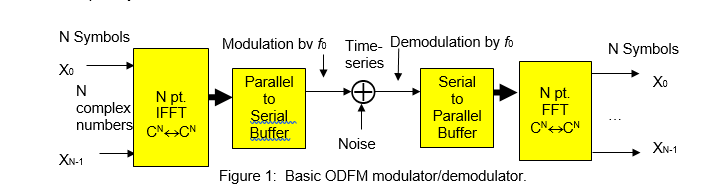# OFDM MATLAB PROJECT 2 Solved

40.00 \$

Category:

## Description

OFDM

Orthogonal frequency division multiplexing (OFDM) is now over 30 years old and belongs to a class of modulation schemes called multi-carrier (MC) system’s. The modulation and demodulation process are generally performed using an FFT and IFFT pair as motivated in Figure1.  The transmission of N complex symbols begins with interpreting each symbol to be transmitted as a complex number that collectively define an N-sample array of complex valued symbols.  The complex array is presented to an N-point IFFT which converts the symbol array into an N-sample complex time-series.  The complex time-series is transmitted and decoded by an FFT located at the receiver that converts the complex time-series back into an array of N complex symbols.Figure 1:  Basic ODFM modulator/demodulator.

Your 64 channel OFDM system imports 16 QPSK symbol arrays of length 64-symbols each..  That is, the system processes 16 sets of[i] 64 QPSK symbols shown below.

(2 bits/symbol)(64 symbols)(16 seta) = 2048 bits (message length)

# MATLAB PROJECT #2

Create (without additive noise) 16 arrays containing 64 randomly chosen QPSK symbols (Figure 2).

2.a.: Run your OFDM simulation measuring  the average error (symbol error rate (SER)) and error variance.

2.b:  Add Gaussian noise to the real and imaginary components of the symbol arrays.  Adjust the noise to produce about 25 dB SNR (verify your SNR). Measure the SER and error variance.

2.c.:  Repeat the 2.b study for a 15 dB SNR study (Verify your SNR).

 16×64 array of samples

Figure 3:  OFDM Simulator

Figure 4: Signal plus noise case.

# MATLAB PROJECT #2

Report the SER and error variance for each case.

Generate Figure 4 graphs for each case.

Project #2 due Oct. 5, 2015.